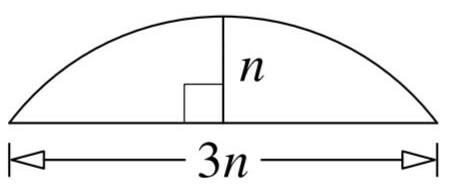# TCS NQT Official Mock Test - 2019 - Quant Advanced

1. A chord of a circle has length of 3n, where n is a positive integer. The segment cutoff my the chord has height n, as shown below. What is the smallest value of n for which the radius of the circle is also a positive integer?2. If n is a positive integer, let s(n) denote the integer obtained by removing the last digit of n and placing it in front. For example, s(731) = 173. What is the smallest positive integer n ending in 6 satisfying s(n) = 4(n)?

3. Of all the nonempty subsets S of {1, 2, 3, 4, 5, 6, 7}, how many do not contain the number |S|, where |S| denotes the number of elements in S? For example, {3, 4} is one such subset, since it does not contain the number 2.

4. A function f satisfies f(0) = 0, f(2n) = f(n), and f(2n+1) = f(n) + 1 for all positive integers n. What is the value of f(2018) ?

5. How many pairs (m, n) of integers satisfy the equation 4^m = n^2 + 15?